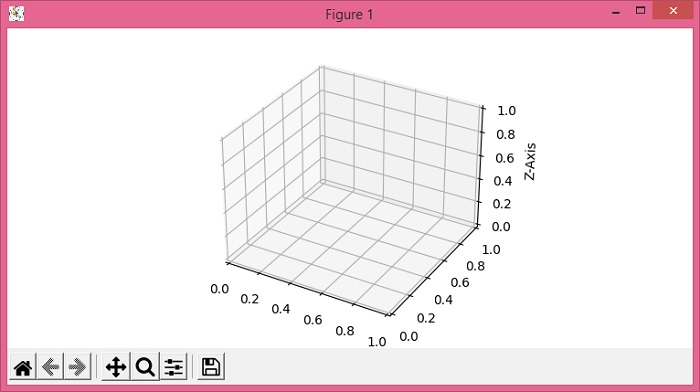# Rotating axes label text in 3D Matplotlib

To rotate axes label text in 3D matplotlib, we can use set_zlabel() method with rotation in the method's argument.

## Steps

• Set the figure size and adjust the padding between and around the subplots.

• Create a new figure or activate an existing figure using figure() method.

• Add a subplot to the current axis with projection="3d".

• Initialize a variable, angle, for an angle.

• Set Z-axis label using set_zlabel() method with a rotation.

• To display the figure, use show() method.

## Example

from matplotlib import pyplot as plt

plt.rcParams["figure.figsize"] = [7.50, 3.50]
plt.rcParams["figure.autolayout"] = True

fig = plt.figure()
plt.show()